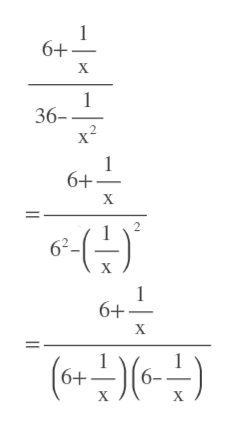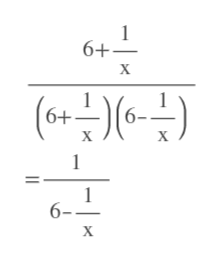the easiest way to simplify the complex rational expression.(6+(1/x))/(36-(1/x^2))

Question

the easiest way to simplify the complex rational expression.

(6+(1/x))/(36-(1/x^2))

Step 1

We have to factor denominator first using a^2 - b^2 = (a+b)(a-b) formula. a=6 and b=1/xhelp_outlineImage Transcriptionclose6+ X 1 36- 6+ X 2 62 1 6+ X 6+ 6- X X fullscreen
Step 2

Cancel (6+1/x). [when x not equ...help_outlineImage Transcriptionclose6+ X ( 6+ 6- X 1 6- X fullscreen

Want to see the full answer?

See Solution

Want to see this answer and more?

Our solutions are written by experts, many with advanced degrees, and available 24/7

See Solution
Tagged in

Other